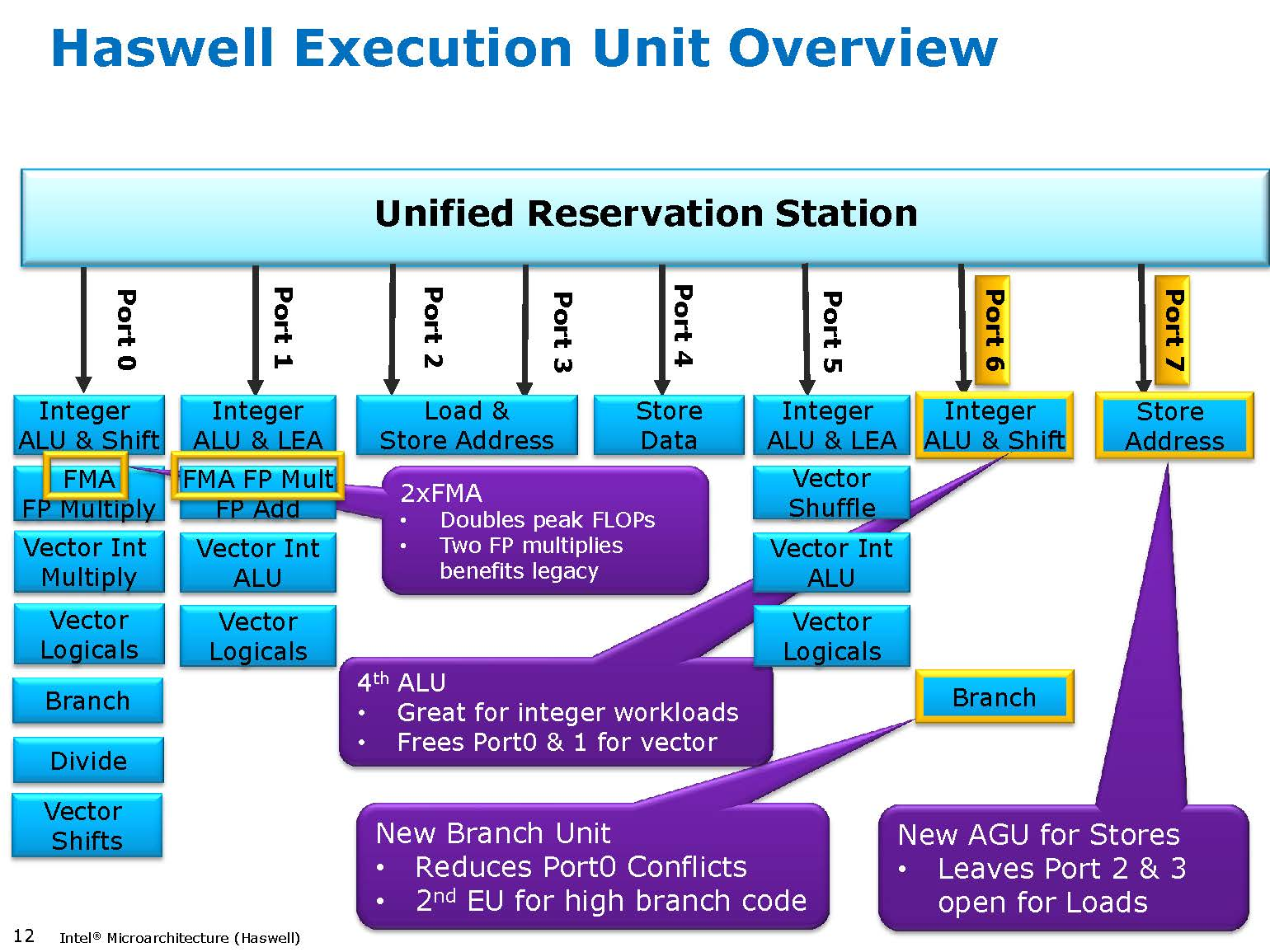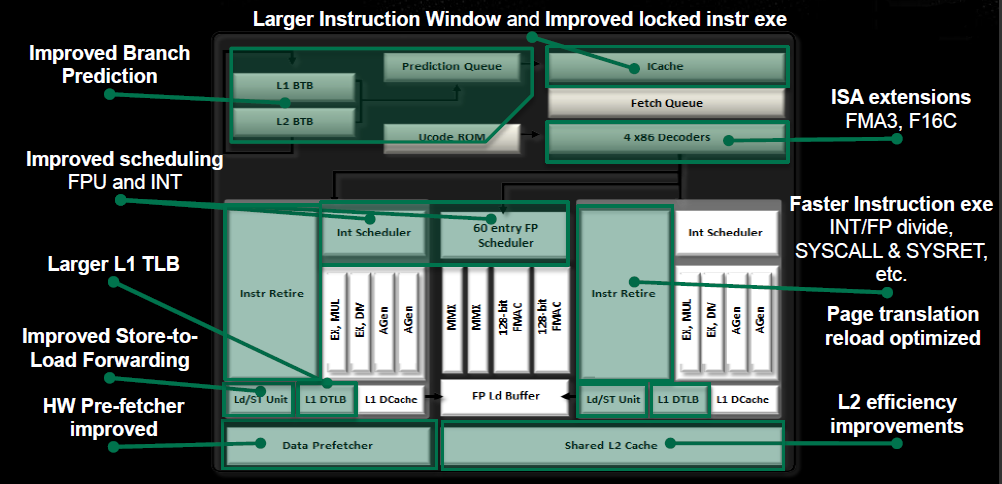# Superscalar Execution

So the 1980's roll on.  Machines get deeper pipelines and better forwarding, until the machines usually initiate a new instruction every clock cycle.  Eventually, even one cycle per instruction (CPI) is not fast enough.  Sadly, one cycle per instruction is the theoretical limit of pipelining--it's the upper bound everybody's trying to reach by avoiding pipeline stalls, hazards, and flushes.

So as the 1990's arrived, designers began adding wide superscalar execution, where multiple instructions start every clock cycle.   Nowadays, the metric is "instructions per cycle" (IPC), which can be substantially more than one.  (Similarly, at some point travel went from gallons per mile, to miles per gallon, which is good!)

To get multiple instructions running at once, you need more than one "execution unit", so more arithmetic can execute at the same time.    For example, 1994's PowerPC 601 could issue one integer, one floating-point, and one load/store instruction every clock cycle.

You can see superscalar effects in the instruction timings of any modern CPU--many instructions simply cost no time at all.  For example, each these assembly programs take the exact same number of nanoseconds on my Pentium 4:

`ret`

(Try this in NetRun now!) -> Takes 4.4ns

```mov eax,8
ret```

(Try this in NetRun now!)  -> Takes 4.4ns

```mov ecx,7
mov eax,8
ret```

(Try this in NetRun now!)  -> Takes 4.4ns

```mov ecx,7
mov eax,ecx
ret```

(Try this in NetRun now!)  -> Takes 4.4ns

```mov ecx,7
ret```

(Try this in NetRun now!)  -> Takes 4.4ns

```mov ecx,7
imul eax,ecx
ret```

(Try this in NetRun now!)  -> Takes 4.4ns

```add ecx,7
imul eax,ecx
ret```

(Try this in NetRun now!)  -> Takes 4.4ns

Yet this one is actually slower by two clock cycles:

```mov ecx,2
mov eax,3
mov edx,4
ret```

(Try this in NetRun now!)

The limiting factor for this particular instruction sequence seems to just be the number of instructions--the Pentium 4's control unit can't handle three writes per clock cycle.

You can avoid the substantial call/return overhead and see a little more timing detail using a tight loop like this one:

```mov ecx,1000
start:
dec ecx
jne start
ret```

(Try this in NetRun now!)

This takes about two clocks to go around each time.   You can even add one more "add" instructions without changing the loop time (ah, superscalar!).  Because the Pentium 4 uses both rising and trailing edges of the clock, a 2.8GHz Pentium 4 (like the main NetRun machine) can take half-integer multiples of the clock period, 0.357ns.  So your timings are 0.357ns for one clock, 0.536ns for 1.5 clocks (like the loop above without the "add"), 0.714ns for 2.0 clocks (like the loop above), 0.893ns for 2.5 clocks, etc.

## Data Dependencies & Register Renaming

The biggest limit to superscalar execution, overall, is data dependencies: RAW, WAR, and WAW, also called "hazards."  (Read it!)

 Read now Write now Read future RAR, not a dependency RAW, true dependency Write future WAR, antidependency WAW, output dependency
• WAW (write after write) is where I write a value after you write it.  The machine just has to make sure the correct value ends up in the register, which is my value.  They call this an "output dependency".
• WAR (write after read) is where I write a value after you read it.  The machine just has to make sure you get your value before I destroy it.  They call this an "antidependency".
• RAW (read after write) is the hard one: I read a value after you write it.  This means I need your value, so I really have to wait for you.  They call these "true dependencies".

You can eliminate the fake dependencies WAW and WAR using register renaming (also called Tomasulo's Algorithm): a typical implementation is to hand out a new renamed register for every write operation.  Modern CPUs rename the architectural registers (like rax or rdi) out to a huge set of *hundreds* of possible physical registers.  Also, because most values are passed directly arithmetic-to-arithmetic (with register bypass), today a "register" is basically just an identifier that the arithmetic units use on their internal operand forwarding network for synchronization.

Notice how if there are no writes, there are no dependencies?  Functional programming extremists contend this indicates writes are evil.
Even if we allow writes, there are several interesting transformations you can use to expose and reduce dependencies, such as Software Pipelining.  A naive application of these is unlikely to provide much benefit, since the compiler and CPU can do naive transformations already; but often you can exploit features of the algorithm to improve performance.

## Why this matters for software

Here's the obvious way to compute the factorial of 12:

```int i, fact=1;
for (i=1;i<=12;i++) {
fact*=i;
}
return fact;```

(Try this in NetRun now!)

Here's a modified version where we separately compute even and odd factorials, then multiply them at the end:

```int i, factO=1, factE=1;
for (i=1;i<=12;i+=2) {
factO*=i;
factE*=i+1;
}
return factO*factE;```

(Try this in NetRun now!)

This modification makes the code "superscalar friendly", so it's possible to execute the loop's multiply instructions simultaniously.  Note that this isn't simply a loop unrolling, which gives a net loss of performance, it's a higher-level transformation to expose parallelism in the problem.

 Hardware Obvious Superscalar Savings Discussion Intel 486, 50MHz, 1991 5000ns 5400ns -10% Classic non-pipelined CPU: many clocks/instruction.  The superscalar transform just makes the code slower, because the hardware isn't superscalar. Intel Pentium III, 1133MHz, 2002 59.6ns 50.1ns +16% Pipelined CPU: the integer unit is fully pipelined, so we get one instruction per clock cycle.  The P3 is also weakly superscalar, but the benefit is small. Intel  Pentium 4, 2.8Ghz, 2005 22.6ns 15.0ns +33% Virtually all of the improvement here is due to the P4's much higher clock rate. Intel Q6600, 2.4GHz, 2008 16.7ns 9.4ns +43% Lower clock rate, but fewer pipeline stages leads to better overall performance. Intel Sandy Bridge i5-2400 3.1Ghz 2011 11.8ns 5.3ns +55% Higher clock rate and better tuned superscalar execution.  Superscalar transform gives a substantial benefit--with everything else getting faster, the remaining dependencies become more and more important.

The same trick of unrolling and breaking dependencies works for a variety of tasks.  For example, this simple "add up the elements of an array" loop takes 330ns on my Skylake CPU:

```const int n=1024;
int arr[n];

long foo(void) {
long sum=0;
for (int i=0;i<n;i++) {
sum+=arr[i];
}
return sum;
}```

(Try this in NetRun now!)

Unrolling the loop cuts the time to 245ns:

```const int n=1024;
int arr[n];

long foo(void) {
long sum=0;
for (int i=0;i<n;i+=2) {
sum+=arr[i];
sum+=arr[i+1];
}
return sum;
}```

(Try this in NetRun now!)

We can improve the performance even more, to 196ns, by breaking the "sum" dependency chain into two halves, and only adding them together once after the entire loop:

```const int n=1024;
int arr[n];

long foo(void) {
long sum0=0, sum1=0;
for (int i=0;i<n;i+=2) {
sum0+=arr[i];
sum1+=arr[i+1];
}
return sum0+sum1;
}```

(Try this in NetRun now!)

Repeating this unroll-and-separate approach 8 times results in 144ns:

```const int n=1024;
int arr[n];

long foo(void) {
long sum0=0, sum1=0, sum2=0, sum3=0, sum4=0, sum5=0, sum6=0, sum7=0;
for (int i=0;i<n;i+=8) {
sum0+=arr[i];
sum1+=arr[i+1];
sum2+=arr[i+2];
sum3+=arr[i+3];
sum4+=arr[i+4];
sum5+=arr[i+5];
sum6+=arr[i+6];
sum7+=arr[i+7];
}
return ((sum0+sum1)+(sum2+sum3))+((sum4+sum5)+(sum6+sum7));
}```

(Try this in NetRun now!)

Note how we add parenthesis at the end to improve parallelism in the final summation (C++'s default is left to right associativity, which makes a sequential dependency chain through all the adds in the last line).

(We haven't hit SIMD yet, but it's faster yet to use the AVX2 SIMD instruction VPMOVSXDQ to sign-extend 4 ints into 4 longs inside a single SIMD register.)

Unrolling 16 loop iterations is slower than this, since we run out of registers to store the intermediate sum variables.

## The Future

It's really interesting to watch the evolution of modern microarchitectures:

• The Intel 8008, from 1972, has a single shared internal bus used for everything: instruction fetch, execution, memory accesses.  This means it takes many clock cycles per instruction, to interleave access to the bus.
• The Intel 386, from the 1980's, added pipelining.  The big difference is you now have many separate busses across the machine, and need to fetch instructions in big blocks (otherwise fetch interferes with execution memory access).
• The Intel Pentium from early 1990 added superscalar execution, so now there are multiple arithmetic units and a dependency-checking control unit.  Initial versions were very weak, adding a second "V pipe" capable of performing common simple instructions (mov, add, inc/dec, push/pop, jmp) alongside the main pipe.
• Since about 2005 we've had several separate processor cores per chip, which requires multicore software.
• A near-modern CPU like the Intel Core2 from the 2000's has renamed registers and an instruction reorder buffer, as described above.There is an inherent tradeoff between complex sophisticated features like out-of-order execution, versus the strength through numbers approach of raw parallelism (such as SIMD or multicore).  Given sufficient software parallelism, out-of-order execution may not be worth the silicon area, and so may die out along with sequential software.  However, some problems don't have much inherent parallelism, and so a mix of fewer more sophisticated cores (CPU) and many simpler cores (GPU) may be the approach of the future.

CS 441 Lecture Note, 2015, Dr. Orion LawlorUAF Computer Science Department.